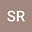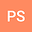••Momentum transport by boundary-layer turbulence causes a weak synoptic-scale vertical motion. The classical textbook solution for the strength of this Ekman pumping depends on the curl of the surface momentum flux. In this study a new solution for Ekman pumping for low Rossby number flow is derived. In particular, the surface momentum flux is parameterized with a commonly used bulk drag formula. This step reveals that the strength of Ekman pumping is bounded. A maximum value is found if the angle between the near-surface wind and the geostrophic wind is 45$\degree$. The weakening of Ekman pumping for enhanced turbulent friction can be simply explained from the fact that an enhanced turbulent drag will reduce the horizontal wind. This may eventually diminish its capacity for large-scale convergence of divergence. As momentum transport is parameterized in large-scale models, the analysis is relevant for the understanding and interpretation of the evolution of synoptic-scale vertical motions as predicted by such models.